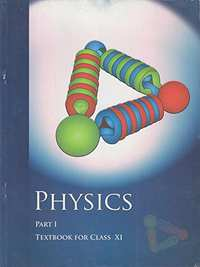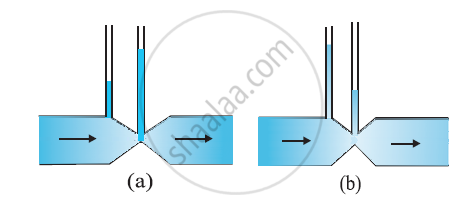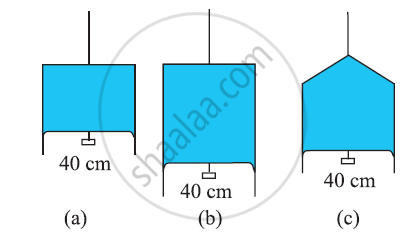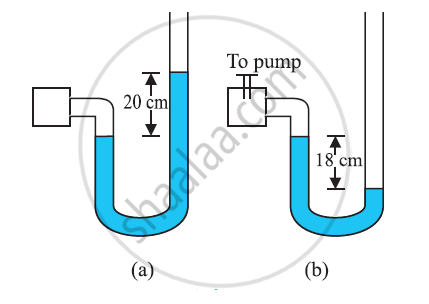Share

# NCERT solutions for Class 11 Physics Textbook chapter 10 - Mechanical Properties of Fluids [Latest edition]

Textbook page

#### Chapters## Chapter 10: Mechanical Properties of Fluids

#### NCERT solutions for Class 11 Physics Textbook Chapter 10 Mechanical Properties of Fluids [Pages 268 - 271]

Q 1.1 | Page 268

Explain why The blood pressure in humans is greater at the feet than at the brain

Q 1.2 | Page 268

Explain why Atmospheric pressure at a height of about 6 km decreases to nearly half of its value at the sea level, though the height of the atmosphere is more than 100 km

Q 1.3 | Page 268

Explain why Hydrostatic pressure is a scalar quantity even though the pressure is force divided by area.

Q 2.1 | Page 268

Explain why The angle of contact of mercury with glass is obtuse, while that of water with glass is acute

Q 2.2 | Page 268

Explain why Water on a clean glass surface tends to spread out while mercury on the same surface tends to form drops. (Put differently, water wets glass while mercury does not.)

Q 2.3 | Page 268

Explain why Surface tension of a liquid is independent of the area of the surface

Q 2.4 | Page 268

Explain why Water with detergent dissolved in it should have small angles of contact.

Q 2.5 | Page 268

Explain why A drop of liquid under no external forces is always spherical in shape

Q 3.1 | Page 268

Fill in the blanks using the word(s) from the list appended with each statement

Surface tension of liquids generally . . . with temperatures (increases / decreases)

Q 3.2 | Page 268

Fill in the blanks using the word(s) from the list appended with each statement

Viscosity of gases. .. with temperature, whereas viscosity of liquids . . . with temperature (increases / decreases)

Q 3.3 | Page 268

Fill in the blanks using the word(s) from the list appended with each statement

For solids with elastic modulus of rigidity, the shearing force is proportional to . . . , while for fluids it is proportional to . .. (shear strain / rate of shear strain)

Q 3.4 | Page 268

Fill in the blanks using the word(s) from the list appended with each statement:

For a fluid in a steady flow, the increase in flow speed at a constriction follows (conservation of mass / Bernoulli’s principle)

Q 3.5 | Page 268

Fill in the blanks using the word(s) from the list appended with each statement

For the model of a plane in a wind tunnel, turbulence occurs at a ... speed for turbulence for an actual plane (greater / smaller)

Q 4.1 | Page 268

Explain why To keep a piece of paper horizontal, you should blow over, not under, it

Q 4.2 | Page 268

Explain why When we try to close a water tap with our fingers, fast jets of water gush through the openings between our fingers

Q 4.3 | Page 268

Explain why The size of the needle of a syringe controls flow rate better than the thumb pressure exerted by a doctor while administering an injection

Q 4.4 | Page 268

Explain why A fluid flowing out of a small hole in a vessel results in a backward thrust on the vessel

Q 4.5 | Page 268

Explain why A spinning cricket ball in air does not follow a parabolic trajectory

Q 5 | Page 268

A 50 kg girl wearing high heel shoes balances on a single heel. The heel is circular with a diameter 1.0 cm. What is the pressure exerted by the heel on the horizontal floor?

Q 6 | Page 269

Toricelli’s barometer used mercury. Pascal duplicated it using French wine of density 984 kg m–3. Determine the height of the wine column for normal atmospheric pressure.

Q 7 | Page 269

A vertical off-shore structure is built to withstand a maximum stress of 109 Pa. Is the structure suitable for putting up on top of an oil well in the ocean? Take the depth of the ocean to be roughly 3 km, and ignore ocean currents.

Q 8 | Page 269

A hydraulic automobile lift is designed to lift cars with a maximum mass of 3000 kg. The area of cross-section of the piston carrying the load is 425 cm2. What maximum pressure would the smaller piston have to bear?

Q 9 | Page 269

A U-tube contains water and methylated spirit separated by mercury. The mercury columns in the two arms are in level with 10.0 cm of water in one arm and 12.5 cm of spirit in the other. What is the specific gravity of spirit?

Q 10 | Page 269

In problem 10.9, if 15.0 cm of water and spirit each are further poured into the respective arms of the tube, what is the difference in the levels of mercury in the two arms? (Specific gravity of mercury = 13.6)

Q 11 | Page 269

Can Bernoulli’s equation be used to describe the flow of water through a rapid in a river? Explain.

Q 12 | Page 269

Does it matter if one uses gauge instead of absolute pressures in applying Bernoulli’s equation? Explain.

Q 13 | Page 269

Glycerine flows steadily through a horizontal tube of length 1.5 m and radius 1.0 cm. If the amount of glycerine collected per second at one end is 4.0 × 10–3 kg s–1, what is the pressure difference between the two ends of the tube? (Density of glycerine = 1.3 × 103 kg m–3 and viscosity of glycerine = 0.83 Pa s). [You may also like to check if the assumption of laminar flow in the tube is correct].

Q 14 | Page 269

In a test experiment on a model aeroplane in a wind tunnel, the flow speeds on the upper and lower surfaces of the wing are 70 m s–1and 63 m s–1 respectively. What is the lift on the wing if its area is 2.5 m2? Take the density of air to be 1.3 kg m–3.

Q 15 | Page 269

Figures (a) and (b) refer to the steady flow of a (non-viscous) liquid. Which of the two figures is incorrect? Why?Q 16 | Page 269

The cylindrical tube of a spray pump has a cross-section of 8.0 cm2 one end of which has 40 fine holes each of diameter 1.0 mm. If the liquid flow inside the tube is 1.5 m min–1, what is the speed of ejection of the liquid through the holes?

Q 17 | Page 269

A U-shaped wire is dipped in a soap solution and removed. The thin soap film formed between the wire and the light slider supports a weight of 1.5 × 10–2 N (which includes the small weight of the slider). The length of the slider is 30 cm. What is the surface tension of the film?

Q 18 | Page 269

Figure  (a) shows a thin liquid film supporting a small weight = 4.5 × 10–2 N. What is the weight supported by a film of the same liquid at the same temperature in Fig. (b) and (c)? Explain your answer physically.Q 19 | Page 270

What is the pressure inside the drop of mercury of radius 3.00 mm at room temperature? The surface tension of mercury at that temperature (20°C) is 4.65 × 10–1 N m–1. The atmospheric pressure is 1.01 × 105 Pa. Also give the excess pressure inside the drop

Q 20 | Page 270

What is the excess pressure inside a bubble of soap solution of radius 5.00 mm, given that the surface tension of soap solution at the temperature (20 °C) is 2.50 × 10–2 N m–1? If an air bubble of the same dimension were formed at depth of 40.0 cm inside a container containing the soap solution (of relative density 1.20), what would be the pressure inside the bubble? (1 atmospheric pressure is 1.01 × 105 Pa).

Q 21 | Page 270

A tank with a square base of area 1.0 m2 is divided by a vertical partition in the middle. The bottom of the partition has a small-hinged door of area 20 cm2. The tank is filled with water in one compartment, and an acid (of relative density 1.7) in the other, both to a height of 4.0 m. compute the force necessary to keep the door close.

Q 22 | Page 270

A manometer reads the pressure of a gas in an enclosure as shown in Figure (a) When a pump removes some of the gas, the manometer reads as in Figure (b) The liquid used in the manometers is mercury and the atmospheric pressure is 76 cm of mercury.

(a) Give the absolute and gauge pressure of the gas in the enclosure for cases (a) and (b), in units of cm of mercury.

(b) How would the levels change in case (b) if 13.6 cm of water (immiscible with mercury) are poured into the right limb of the manometer? (Ignore the small change in the volume of the gas).Q 23 | Page 270

Two vessels have the same base area but different shapes. The first vessel takes twice the volume of water that the second vessel requires to fill up to a particular common height. Is the force exerted by the water on the base of the vessel the same in the two cases? If so, why do the vessels filled with water to that same height give different readings on a weighing scale?

Q 24 | Page 271

During blood transfusion the needle is inserted in a vein where the gauge pressure is 2000 Pa. At what height must the blood container be placed so that blood may just enter the vein? [Use the density of whole blood from Table 10.1].

Q 25 | Page 271

In deriving Bernoulli’s equation, we equated the work done on the fluid in the tube to its change in the potential and kinetic energy. (a) What is the largest average velocity of blood flow in an artery of diameter 2 × 10–3 m if the flow must remain laminar? (b) Do the dissipative forces become more important as the fluid velocity increases? Discuss qualitatively.

Q 26 | Page 271

(a) What is the largest average velocity of blood flow in an artery of radius 2 × 10–3 m if the flow must remain laminar? (b) What is the corresponding flow rate? (Take viscosity of blood to be 2.084 × 10–3 Pa s).

Q 27 | Page 271

A plane is in level flight at a constant speed and each of its two wings has an area of 25 m2. If the speed of the air is 180 km/h over the lower wing and 234 km/h over the upper wing surface, determine the plane’s mass. (Take air density to be 1 kg m–3).

Q 28 | Page 271

In Millikan’s oil drop experiment, what is the terminal speed of an uncharged drop of radius 2.0 × 10–5 m and density 1.2 × 103 kg m–3? Take the viscosity of air at the temperature of the experiment to be 1.8 × 10–5 Pa s. How much is the viscous force on the drop at that speed? Neglect buoyancy of the drop due to air.

Q 29 | Page 271

Mercury has an angle of contact equal to 140° with soda lime glass. A narrow tube of radius 1.00 mm made of this glass is dipped in a trough containing mercury. By what amount does the mercury dip down in the tube relative to the liquid surface outside? Surface tension of mercury at the temperature of the experiment is 0.465 N m–1. Density of mercury = 13.6 × 103 kg m–3

Q 30 | Page 271

Two narrow bores of diameters 3.0 mm and 6.0 mm are joined together to form a U-tube open at both ends. If the U-tube contains water, what is the difference in its levels in the two limbs of the tube? Surface tension of water at the temperature of the experiment is 7.3 × 10–2 N m–1. Take the angle of contact to be zero and density of water to be 1.0 × 103 kg m–3 (g = 9.8 m s–2)

Q 31.1 | Page 271

It is known that density ρ of air decreases with height as 0^(e^(y/y_0)) Whererho_0 1.25 kg m–3 is the density at sea level, and y0 is a constant. This density variation is called the law of atmospheres. Obtain this law assuming that the temperature of atmosphere remains a constant (isothermal conditions). Also, assume that the value of gremains constant

Q 31.2 | Page 271

A large He balloon of volume 1425 m3 is used to lift a payload of 400 kg. Assume that the balloon maintains constant radius as it rises. How high does it rise? [Take y0= 8000 m and rho_"He"= 0.18 kg m–3].

## Chapter 10: Mechanical Properties of Fluids## NCERT solutions for Class 11 Physics Textbook chapter 10 - Mechanical Properties of Fluids

NCERT solutions for Class 11 Physics Textbook chapter 10 (Mechanical Properties of Fluids) include all questions with solution and detail explanation. This will clear students doubts about any question and improve application skills while preparing for board exams. The detailed, step-by-step solutions will help you understand the concepts better and clear your confusions, if any. Shaalaa.com has the CBSE Class 11 Physics Textbook solutions in a manner that help students grasp basic concepts better and faster.

Further, we at Shaalaa.com provide such solutions so that students can prepare for written exams. NCERT textbook solutions can be a core help for self-study and acts as a perfect self-help guidance for students.

Concepts covered in Class 11 Physics Textbook chapter 10 Mechanical Properties of Fluids are Introduction to Fluid Machanics, Archimedes' Principle, Stokes' Law, Equation of Continuity, Torricelli'S Law, Concept of Pressure, Pascal's Law, Variation of Pressure with Depth, Atmospheric Pressure and Gauge Pressure, Hydraulic Machines, STREAMLINE FLOW, Bernoulli’S Principle, Viscosity, Reynolds Number, Surface Tension, Effect of Gravity on Fluid Pressure, Terminal Velocity, Critical Velocity, Excess of Pressure Across a Curved Surface.

Using NCERT Class 11 solutions Mechanical Properties of Fluids exercise by students are an easy way to prepare for the exams, as they involve solutions arranged chapter-wise also page wise. The questions involved in NCERT Solutions are important questions that can be asked in the final exam. Maximum students of CBSE Class 11 prefer NCERT Textbook Solutions to score more in exam.

Get the free view of chapter 10 Mechanical Properties of Fluids Class 11 extra questions for Class 11 Physics Textbook and can use Shaalaa.com to keep it handy for your exam preparation

S